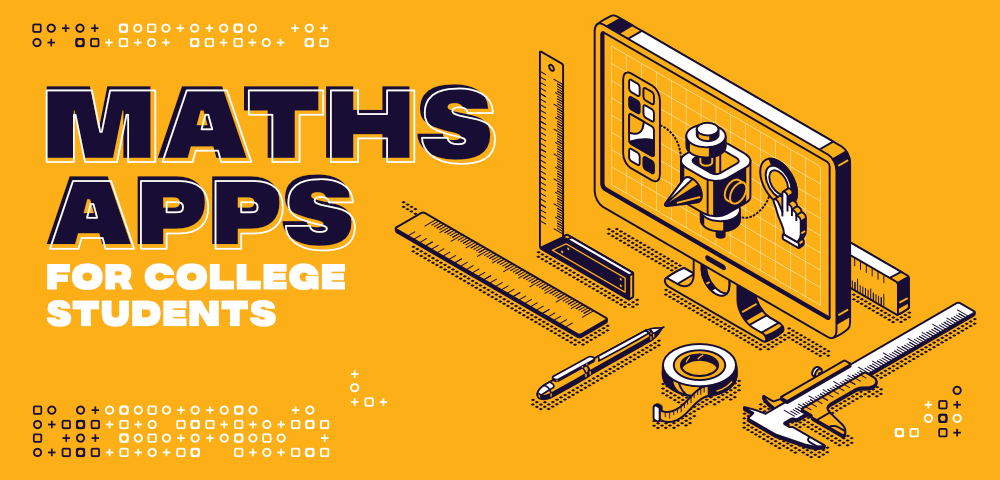Related Articles
Top Maths Apps for College Students in 2020
• Last Updated : 10 Sep, 2020

Maths is like a torture session in school and college! I am sure most of you don’t enjoy Maths and just have to do it to complete your educational requirements. However, Maths can be pretty interesting and engaging if you concentrate. Math problems are almost like puzzles that you have to solve to get the correct answer. But it can be difficult to solve these puzzles and be completely sure you have the correct answer if you are a beginner. That’s why this article details the top Maths apps that will help you in completing Math problems. These apps will double-check your answers, provide you step by step instructions for problem solutions if you are in doubt, plot a graph for any function you want, and much more.Now, are you wondering why am I discussing Maths? Isn’t that just a subject you do in school and then move on after college? No, Maths is a very important part of many specializations such as Computer Science. While Boolean Algebra is used in Logic Gates, Relational Algebra is used in Databases. Number Theory also has multiple applications in Cryptography and Cryptanalysis. And let’s not forget the hot topic these days, Data Science! Mathematical concepts like Linear algebra, Multivariable calculus, Probability Theory, etc. are a foundation stone of fields like Data Science, Artificial Intelligence, Machine Learning, etc.

That is why Maths is so important and it is needed even after high school, especially in Computer Science. So if you are practicing Maths, then all these apps will be extremely useful to you as they will make studying much easier. So, let’s check out these apps now!

### 1. Photomath

Photomath can solve your equations for you and the best thing is you don’t even need to type them out. It uses augmented reality to scan the maths equations written on paper and then find the solutions to these equations for you. However, it’s still a bit weak at analyzing equations written in your handwriting so printed is the best option. Photomath also displaying the answers to these equations in full detail so that you can intuitively follow the steps and arrive at the final solution. While this app is still not that good with quadratic equations and calculus problems it’s the perfect option for algebraic equations and arithmetic problems.

### 2. Graphing Calculator

Sometimes its much easier to be able to see the graphs of functions for various Maths problems. For example, it’s very easy to note where the function crosses the x-axis on a graph rather than computing it. In situations like these, the Graphing Calculator by MathLab is extremely handy. You can also use it for many other calculations such as finding derivatives and integrals and simplifying mathematical expressions. While you may not be able to do this in exams, it will still be very helpful while practicing and double-checking your answers.

### 3. Maths Solver

Maths Solver is an app created by Microsoft that can help in solving mathematical equations such as arithmetic equations, trigonometry, linear algebra, calculus, statistics, etc. This app accomplishes this using an Artificial Intelligence based maths solver. In addition to this, Maths Solver provides step-by-step explanations for its solutions. You can also obtain interactive graphs relating to your equations and any similar problems that are available from the internet if you want to do more practice. After all, in maths, the more you practice, the better it is!

### 4. iMathematics

iMathematics can solve various maths equations along with providing formulas, theorems, definitions, and properties that you can easily learn. There are also other facilities like the Graphic Calculator, Matrices Solver, Math Glossary, etc. The iMathematics Pro, which is the paid version can do even more and perform complex mathematical equations. The only disadvantage of this app is that it cannot read maths equations from paper-like Photomath and you have to type them out.

### 5. Unit Converter

Confused how many millimeters there are in centimeters or how many inches in a foot? It happens to all of all and it’s hard to remember these conversions anyway. That’s where the unit converter comes to your help. It can convert any value to another such as heat, currency, magnetism, data, fluid, light, energy, power, temperature, etc. Not only maths, but this is also extremely helpful if you are studying other subjects like computer science, physics, chemistry, etc. And if you are a tourist needing to convert rupees to dollars in real-time currency rate, this is your best friend!

### Conclusion

Maths is not just a standalone subject but also the foundation of many advanced fields. Therefore, it is very important that you are proficient in Maths. And even if you are not an expert, at least you should not hate this subject! So go through these apps, and select the one you like the most. You can use that to practice Maths so that you get better and better and don’t fear Maths anymore. Because this subject can be your best friend and help you tremendously in the future, especially if you take up Computer Science and its advanced specializations.My Personal Notes arrow_drop_up